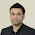Thoughtworks interview question

You decided to give up on earth after the latest financial collapse left 99.99% of the earth's population with 0.01% of the wealth. Luckily, with the scant sum of money that is left in your account, you are able to afford to rent a spaceship, leave earth, and fly all over the galaxy to sell common metals and dirt (which apparently is worth a lot).Buying and selling over the galaxy requires you to convert numbers and units, and you decided to write a program to help you.The numbers used for intergalactic transactions follows similar convention to the roman numerals and you have painstakingly collected the appropriate translation between them.Roman numerals are based on seven symbols.

SymbolValue
• I 1
• V 5
• X 10
• L 250
• C 100
• D 500
• M 1,000
Numbers are formed by combining symbols together and adding the values. For example, MMVI is 1000 + 1000 + 5 + 1 = 2006. Generally, symbols are placed in order of value, starting with the largest values. When smaller values precede larger values, the smaller values are subtracted from the larger values, and the result is added to the total. For example MCMXLIV = 1000 + (1000 - 100) + (50 - 10) + (5 - 1) = 1944. The symbols "I", "X", "C", and "M" can be repeated three times in succession, but no more. (They may appear four times if the third and fourth are separated by a smaller value, such as XXXIX.) "D", "L", and "V" can never be repeated. "I" can be subtracted from "V" and "X" only. "X" can be subtracted from "L" and "C" only. "C" can be subtracted from "D" and "M" only. "V", "L", and "D" can never be subtracted. Only one small-value symbol may be subtracted from any large-value symbol. A number written in Arabic numerals can be broken into digits. For example, 1903 is composed of 1, 9, 0, and 3. To write the Roman numeral, each of the non-zero digits should be treated separately. In the above example, 1,000 = M, 900 = CM, and 3 = III. Therefore, 1903 = MCMIII. -- Source: Wikipedia (http://en.wikipedia.org/wiki/Roman_numerals)Input to your program consists of lines of text detailing your notes on the conversion between intergalactic units and roman numerals. You are expected to handle invalid queries appropriately. Test input:

glob is I
prok is V
pish is X
tegj is L
glob glob Silver is 34 Credits

glob prok Gold is 57800 Credits
pish pish Iron is 3910 Credits
how much is pish tegj glob glob ?
how many Credits is glob prok Silver ?
how many Credits is glob prok Gold ?
how many Credits is glob prok Iron ?
how much wood could a woodchuck chuck if a woodchuck could chuck wood ?

Test Output:

pish tegj glob glob is 42
glob prok Silver is 68 Credits
glob prok Gold is 57800 Credits
glob prok Iron is 782 Credits
I have no idea what you are talking about

1.I doubt the value of L is 50 or 250..?

1.True..

It should be 250 right ?

2.True..

It should be 250 right ?

3.Question is wrong, L should be 50.

2.Perhaps, question has been changed by now

3.What r the values 4 gold silver n iron

4.What r the values 4 gold silver n iron